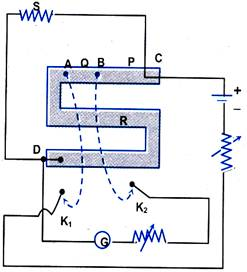Click to Chat

1800-1023-196

+91-120-4616500

CART 0

• 0

MY CART (5)

Use Coupon: CART20 and get 20% off on all online Study Material

ITEM
DETAILS
MRP
DISCOUNT
FINAL PRICE
Total Price: Rs.

There are no items in this cart.
Continue Shopping• Complete Physics Course - Class 11
• OFFERED PRICE: Rs. 2,968
• View Details

```Measurement of an unknown resistance using a Post Office Box

A Post Office Box can also be used to measure an unknown resistance. It is a Wheatstone Bridge with three arms P, Q and R; while the fourth arm(s) is the unknown resistance. P and Q are known as the ratio arms while R is known as the rheostat arm.

At balance, the unknown resistance

S = (P/Q) R                                                           …… (1)

The ratio arms are first adjusted so that they carry 100 W each. The resistance in the rheostat arm is now adjusted so that the galvanometer deflection is in one direction. If R = R0 (ohm) and
R = R0 + 1 (ohm) are the resistance in rheostat arm, for which the deflection in galvanometer is in opposite direction, then it implies that the unknown resistance ‘s’ lies between R0 & (R0 + 1) ohm.Alt txt: resistance-using-post-office-box

Now, the resistance in P and Q are made 100 W and 1000 W, respectively, and the process is repeated. Equation (1) is used to compute S. The ratio P/Q is progressively made 1:10, and then 1:100. Thus, the resistance S can be accurately measured.

Errors

The major sources of error are the connecting wires, unclear resistance plugs, change in resistance due to Joule heating, and the insensitivity of the Wheatstone bridge

These errors may be removed by using thick connecting wires, clean plugs, keeping the circuit on for very brief periods (to avoid Joule heating) and calculating the sensitivity.

In order that the sensitivity is maximum, the resistance in the arm P is kept close to the value of the resistance S.

Illustration:

Resistance of a conductor is 1.72 W at a temperature of 20°C. Find the resistance at 0oC and 100°C. Given the coefficient of resistivity is
a = 0.00393/oC.

Solution:

R = R0 (1 + aDT)

R = 1.72 W (1 + 0.00393) × (0°C – 20°C) = 1.58 W

At T = 100°C

R = 1.72 W [1 + 0.00393) × (100°C – 20°C)] = 2.26 W

To read more, Buy study materials of Current Electricity comprising study notes, revision notes, video lectures, previous year solved questions etc. Also browse for more study materials on Physics here.
```### Course Features

• 101 Video Lectures
• Revision Notes
• Previous Year Papers
• Mind Map
• Study Planner
• NCERT Solutions
• Discussion Forum
• Test paper with Video Solution